## Getting started

A polygon is a plane figure that is described by a finite number of straight-line segments connected to form a closed polygonal chain (Singer, 1993)1.

Given the above, we may conclude that image objects can be expressed as polygons with n vertices. pliman has a set of useful functions (draw_*()) to draw common shapes such as circles, squares, triangles, rectangles and n-tagons. Another group of poly_*() functions can be used to analyze polygons. Let’s start with a simple example, related to the area and perimeter of a square.

library(pliman)
#> The legacy packages maptools, rgdal, and rgeos, underpinning the sp package,
#> which was just loaded, will retire in October 2023.
#> Please refer to R-spatial evolution reports for details, especially
#> https://r-spatial.org/r/2023/05/15/evolution4.html.
#> It may be desirable to make the sf package available;
#> package maintainers should consider adding sf to Suggests:.
#> The sp package is now running under evolution status 2
#>      (status 2 uses the sf package in place of rgdal)
#> |==========================================================|
#> | Tools for Plant Image Analysis (pliman 2.0.1)            |
#> | Author: Tiago Olivoto                                    |
#> | Type citation('pliman') to know how to cite pliman     |
#> | Visit 'http://bit.ly/pkg_pliman' for a complete tutorial |
#> |==========================================================|
square <- draw_square(side = 1)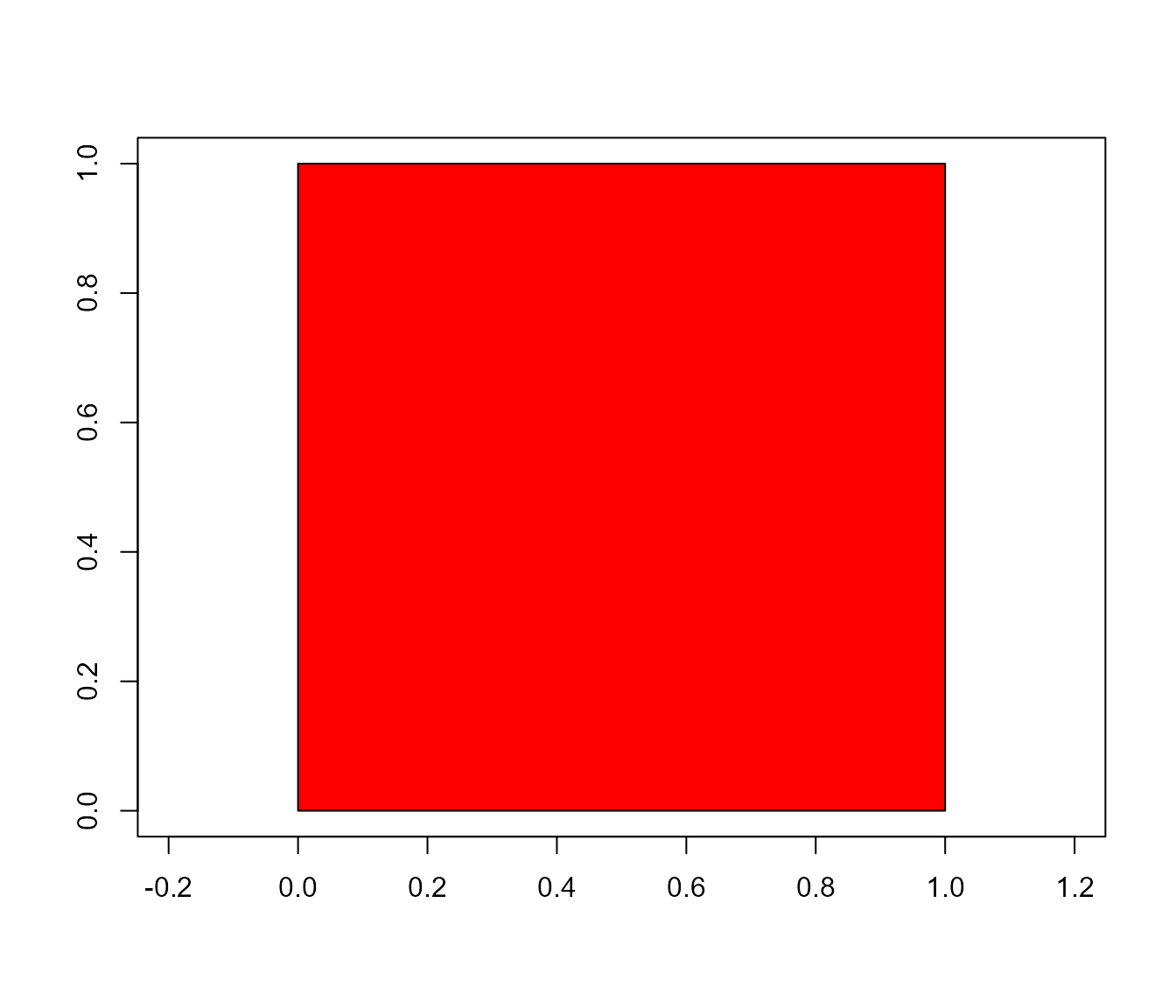poly_area(square)
#>  1
poly_perimeter(square)
#>  3

Now, Let’s see what happens when we start with a hexagon and increase the number of sides up to 1000.

shapes <- list(side6 <- draw_n_tagon(6, plot = FALSE),
side12 <- draw_n_tagon(12, plot = FALSE),
side24 <- draw_n_tagon(24, plot = FALSE),
side100 <- draw_n_tagon(100, plot = FALSE),
side500 <- draw_n_tagon(500, plot = FALSE),
side100 <- draw_n_tagon(1000, plot = FALSE))
plot_polygon(shapes, merge = FALSE)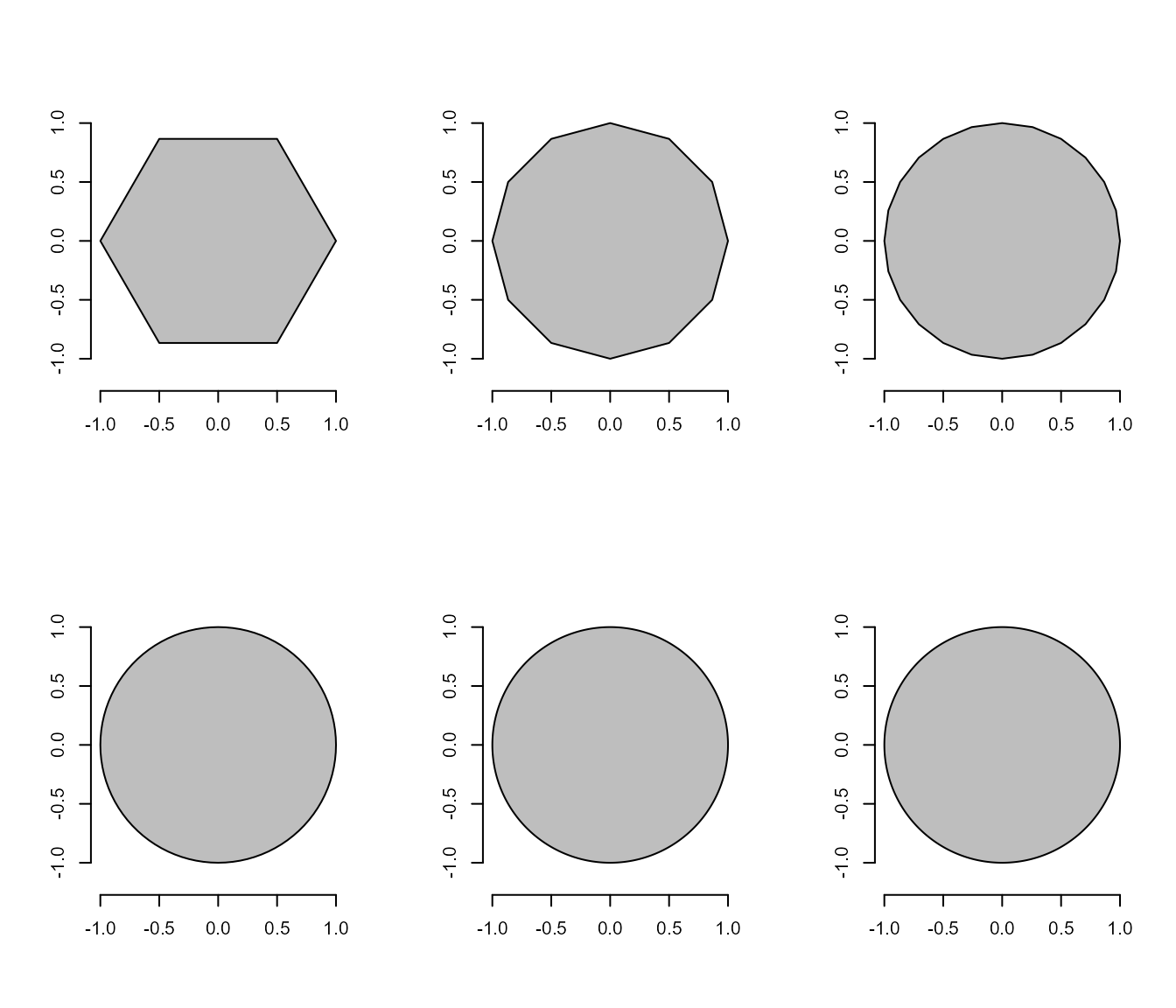poly_area(shapes)
#>  2.598076 3.000000 3.105829 3.139526 3.141510 3.141572
poly_perimeter(shapes)
#>  5.000000 5.694019 6.004205 6.219330 6.270578 6.276892

Note that when $$n \to \infty$$, the sum of sides becomes the circumference of the circle, given by $$2\pi r$$, and the area becomes $$\pi r^2$$. This is cool, but pliman is mainly designed to analyze plant image analysis. So, why would we use polygons? Let’s see how we can use these functions to obtain useful information.

link <- "https://raw.githubusercontent.com/TiagoOlivoto/tiagoolivoto/master/static/tutorials/pliman_lca/imgs/leaves.jpg"
leaves <- image_import(link, plot = TRUE)
cont <- object_contour(leaves, watershed = FALSE, index = "HI")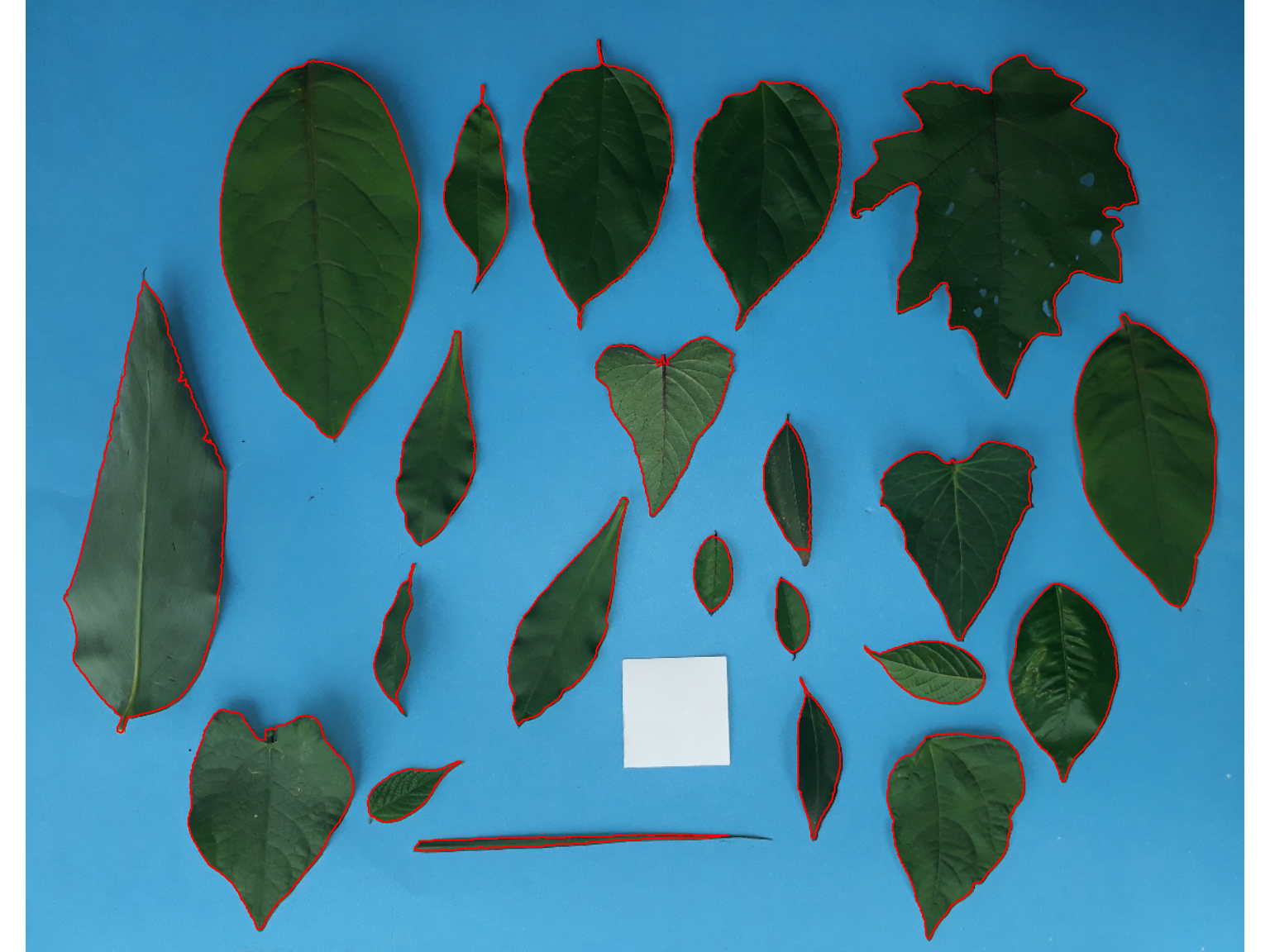# plotting the polygon
plot_polygon(cont)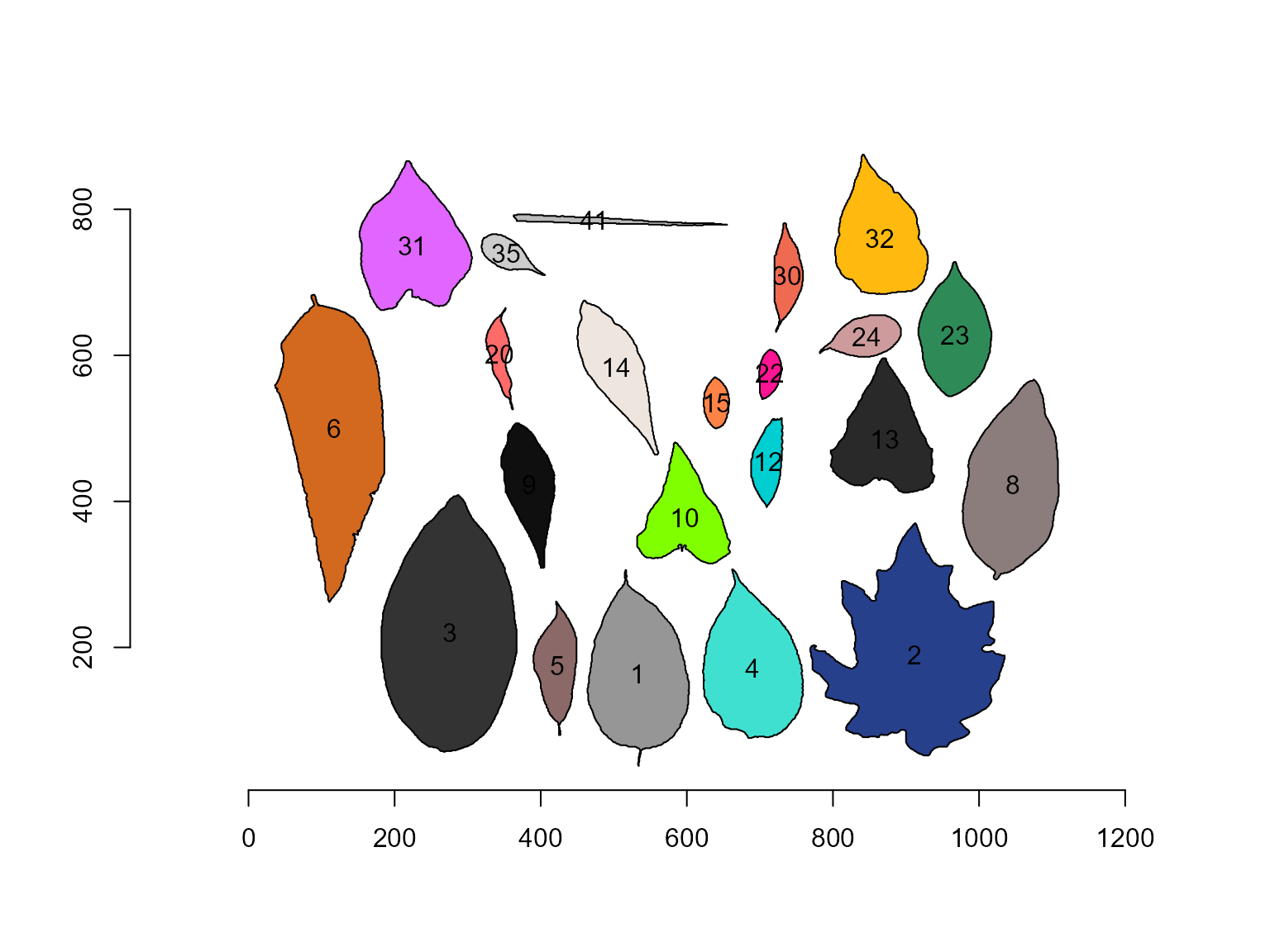## Object measures

Nice! We can use the contour of any object to obtain useful information related to its shape. To reduce the amount of output, I will only use five samples: 2, 4, 13, 24, and 35.

cont <- cont[c("2", "4", "13", "24", "41")]
plot_polygon(cont)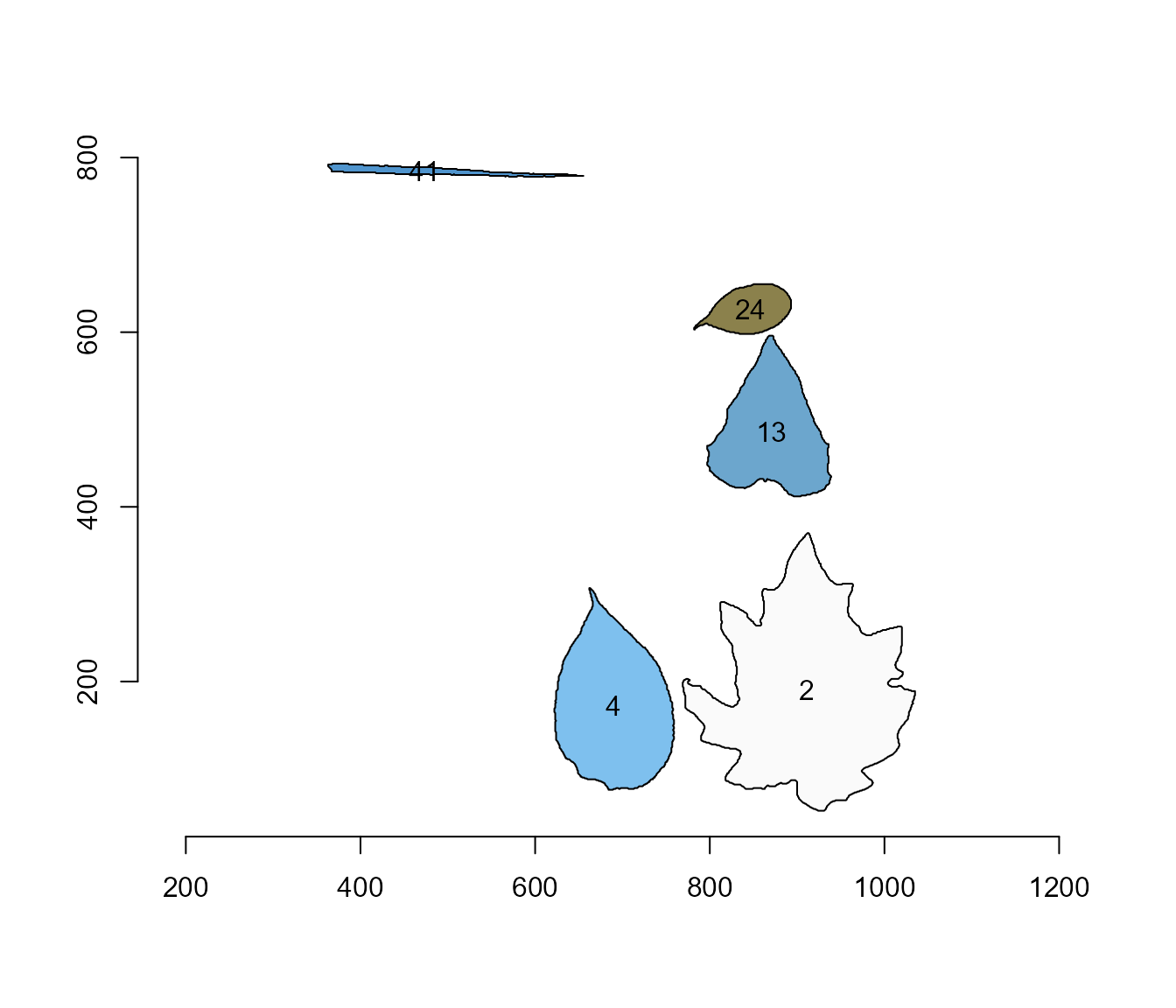In the current version of pliman, you will be able to compute the following measures. For more details, see Chen & Wang (2005)2, Claude (2008)3, and Montero et al. 20094.

### Area

The area of a shape is computed using Shoelace Formula (Lee and Lim, 2017)5, as follows

$A=\frac{1}{2}\left|\sum_{i=1}^{n}\left(x_{i} y_{i+1}-x_{i+1}y_{i}\right)\right|$

poly_area(cont)
#>  45075.0 20793.5 15183.5  4144.0  1688.0

### Perimeter

The perimeter is computed as the sum of the euclidean distance between every point of a shape. The distances can be obtained with poly_distpts().

poly_perimeter(cont)
#>         2         4        13        24        41
#> 1290.1413  630.7128  565.7422  291.5929  603.1543

# perimeter of a circle with radius equals to 2
circle <- draw_circle(radius = 2, plot = FALSE)
poly_perimeter(circle)
#>  12.56635

# check the result
2*pi*2
#>  12.56637

The radius of a pixel in the object contour is computed as its distance to the object centroid (also called as ‘center of mass’). These distances can be obtained with poly_centdist(). The average, maximum and minimum radius can be obtained.

dists <- poly_centdist(cont)

mean_list(dists)
#>         2         4        13        24        41
#> 119.55450  85.22870  72.13064  39.10193  73.70601
min_list(dists)
#>         2         4        13        24        41
#> 68.938361 62.146291 48.670983 25.480450  2.638939
max_list(dists)
#>         2         4        13        24        41
#> 171.20105 129.75938 108.46217  61.56336 147.04645
sd_list(dists)
#>        2        4       13       24       41
#> 20.94407 16.84946 14.62283 10.26425 42.27660

# average radius of the circle above
poly_centdist(circle) |> mean_list()
#>  1.999998

### Length and width

The length and width of an object are computed with poly_lw() as the difference between the maximum and minimum of x and y coordinates after the object has been aligned with poly_align().

aligned <- poly_align(cont)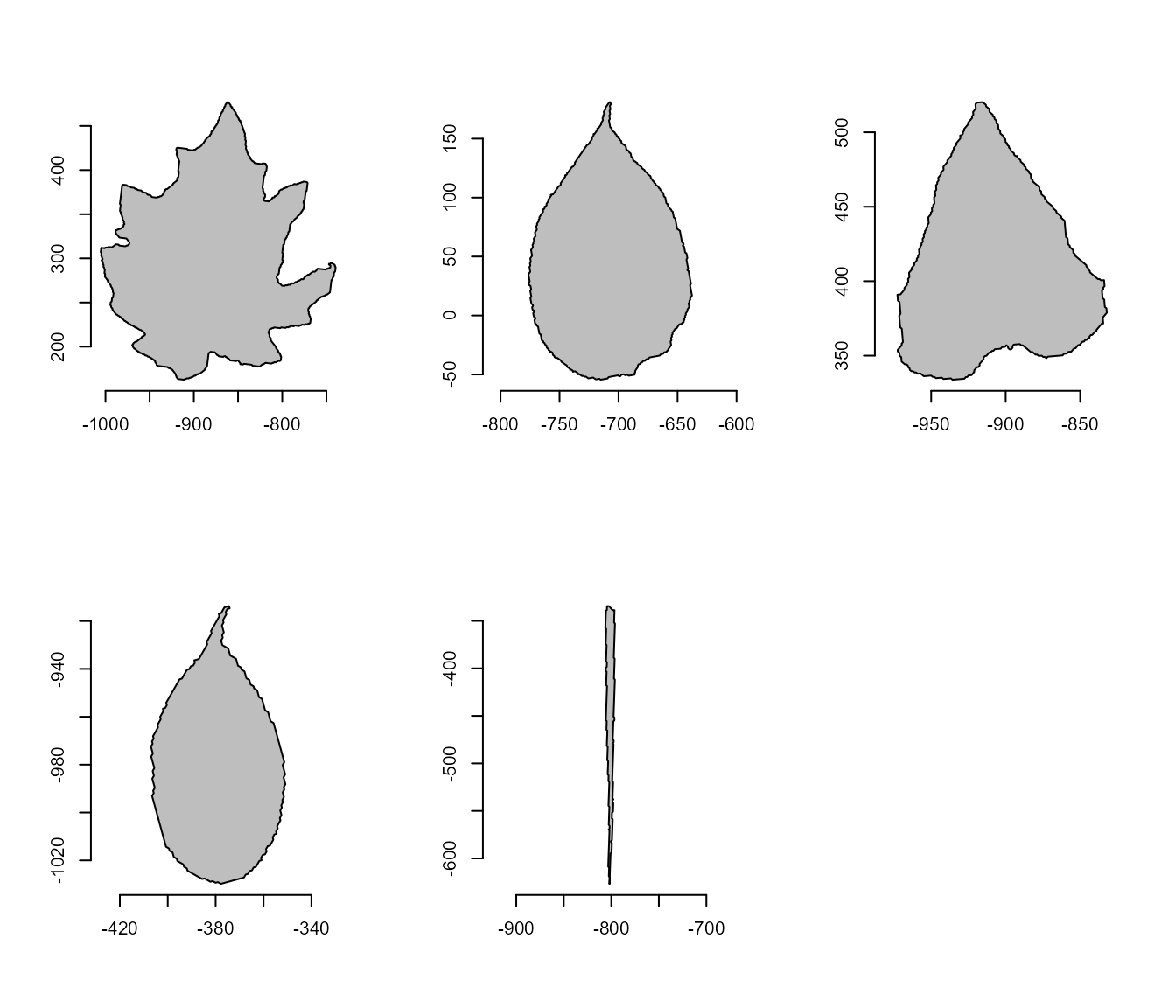# compute length and width
poly_lw(cont)
#>        length     width
#> [1,] 314.3777 265.87632
#> [2,] 235.4453 138.61494
#> [3,] 186.3901 140.53457
#> [4,] 116.0408  56.15904
#> [5,] 292.2781  10.17229

### Circularity, eccentricity, caliper, and elongation

Circularity measure (Montero et al. 2009)6 is also called shape compactness, or roundness measure of an object. It is given by $$C = P^2 / A$$, where $$P$$ is the perimeter and $$A$$ is the area of the object.

poly_circularity(cont)
#>         2         4        13        24        41
#>  36.92656  19.13091  21.07974  20.51796 215.51845

Since the above measure is dependent on the scale, the normalized circularity can be used. In this case, it is assumed to be unity for a circle. This measure is invariant under translation, rotation, scaling transformations, and dimensionless. It is given by: $$Cn = P^2 / 4 \pi A$$

poly_circularity_norm(cont)
#>          2          4         13         24         41
#> 0.34030714 0.65686211 0.59613497 0.61245704 0.05830763

# normalized circularity for different shapes
draw_square(plot = FALSE) |> poly_circularity_norm()
#>  1.396263
draw_circle(plot = FALSE) |> poly_circularity_norm()
#>  0.9999967

poly_circularity_haralick() computes the Haralick’s circularity (CH). The method is based on the computation of all the Euclidean distances from the object centroid to each boundary pixel. With this set of distances, the mean ($$m$$) and the standard deviation ($$s$$) are computed. These statistical parameters are used on a ratio that calculates the circularity, CH, of a shape, as $$CH = m/sd$$

poly_circularity_haralick(cont)
#>        2        4       13       24       41
#> 5.708275 5.058245 4.932743 3.809527 1.743423

poly_convexity() Computes the convexity of a shape using a ratio between the perimeter of the convex hull and the perimeter of the polygon.

poly_convexity(cont)
#>         2         4        13        24        41
#> 0.6435196 0.8850799 0.7579630 0.9099463 0.7222449

poly_eccentricity() Computes the eccentricity of a shape using the ratio of the eigenvalues (inertia axes of coordinates).

poly_eccentricity(cont)
#>             [,1]
#> [1,] 0.833397171
#> [2,] 0.421770889
#> [3,] 0.599180391
#> [4,] 0.297943286
#> [5,] 0.001438398

poly_elongation() Computes the elongation of a shape as 1 - width / length

poly_elongation(cont)
#>           [,1]
#> [1,] 0.1542773
#> [2,] 0.4112648
#> [3,] 0.2460192
#> [4,] 0.5160407
#> [5,] 0.9651965

poly_caliper() Computes the caliper (Also called the Feret’s diameter).

poly_caliper(cont)
#>        2        4       13       24       41
#> 317.5106 229.5125 187.4807 115.1347 253.3338

Users can use the function poly_measures() to compute most of the object measures in a single call.

(measures <- poly_measures(cont))
#> 2   1 911.0558 190.1729 45075.0   57540 1290.1413   119.55450  68.938361
#> 4   2 689.1227 171.8705 20793.5   21924  630.7128    85.22870  62.146291
#> 13  3 870.4033 485.5803 15183.5   16554  565.7422    72.13064  48.670983
#> 24  4 846.2110 625.7005  4144.0    4433  291.5929    39.10193  25.480450
#> 41  5 472.9797 784.6601  1688.0    2167  603.1543    73.70601   2.638939
#> 2   171.20105  20.94407     2.483393 239.10899 137.876721 342.4021 314.3777
#> 4   129.75938  16.84946     2.087967 170.45741 124.292582 259.5188 235.4453
#> 13  108.46217  14.62283     2.228477 144.26129  97.341966 216.9243 186.3901
#> 24   61.56336  10.26425     2.416102  78.20386  50.960900 123.1267 116.0408
#> 41  147.04645  42.27660    55.721805 147.41202   5.277878 294.0929 292.2781
#>      length     width  solidity convexity elongation circularity
#> 2  314.3777 265.87632 0.7833681 0.6435196  0.1542773    36.92656
#> 4  235.4453 138.61494 0.9484355 0.8850799  0.4112648    19.13091
#> 13 186.3901 140.53457 0.9172103 0.7579630  0.2460192    21.07974
#> 24 116.0408  56.15904 0.9348071 0.9099463  0.5160407    20.51796
#> 41 292.2781  10.17229 0.7789571 0.7222449  0.9651965   215.51845
#>    circularity_haralick circularity_norm eccentricity       pcv
#> 2              5.708275       0.34030714  0.833397171 2.6693543
#> 4              5.058245       0.65686211  0.421770889 0.8422071
#> 13             4.932743       0.59613497  0.599180391 1.1910148
#> 24             3.809527       0.61245704  0.297943286 1.7042851
#> 41             1.743423       0.05830763  0.001438398 0.6421213

If the image resolution is known, then, the measures can be corrected with get_measures(). The image resolution can be obtained using a known distance in the image. In the example, the white square has a side of 5 cm. So, using dpi() the resolution can be obtained. In this case, the dpi is ~50.

(measures_cor <- get_measures(measures, dpi = 50))
#> 2   1 911.0558 190.1729 116.32235 148.49003  65.53918     6.07337    3.50207
#> 4   2 689.1227 171.8705  53.66054  56.57795  32.04021     4.32962    3.15703
#> 13  3 870.4033 485.5803  39.18315  42.71991  28.73970     3.66424    2.47249
#> 24  4 846.2110 625.7005  10.69417  11.43998  14.81292     1.98638    1.29441
#> 41  5 472.9797 784.6600   4.35612   5.59225  30.64024     3.74427    0.13406
#> 2     8.69701   1.06396      0.12616  12.14674  7.00414 17.39403 15.97039
#> 4     6.59178   0.85595      0.10607   8.65924  6.31406 13.18355 11.96062
#> 13    5.50988   0.74284      0.11321   7.32847  4.94497 11.01976  9.46862
#> 24    3.12742   0.52142      0.12274   3.97276  2.58881  6.25484  5.89487
#> 41    7.46996   2.14765      2.83067   7.48853  0.26812 14.93992 14.84773
#>      length    width solidity convexity elongation circularity
#> 2  15.97039 13.50652  0.03980   0.64352    0.15428    36.92656
#> 4  11.96062  7.04164  0.04818   0.88508    0.41126    19.13091
#> 13  9.46862  7.13916  0.04659   0.75796    0.24602    21.07974
#> 24  5.89487  2.85288  0.04749   0.90995    0.51604    20.51796
#> 41 14.84773  0.51675  0.03957   0.72224    0.96520   215.51845
#>    circularity_haralick circularity_norm eccentricity     pcv     pcv
#> 2               5.70828          0.34031      0.83340 2.66935 2.66935
#> 4               5.05825          0.65686      0.42177 0.84221 0.84221
#> 13              4.93274          0.59613      0.59918 1.19101 1.19101
#> 24              3.80953          0.61246      0.29794 1.70429 1.70429
#> 41              1.74342          0.05831      0.00144 0.64212 0.64212

## A little bit more!

Some useful functions can be used to manipulate coordinates. In the following example, I will show some features implemented in pliman. Just for simplicity, I will use only object 2.

o2 <- cont[["2"]]
plot_polygon(o2)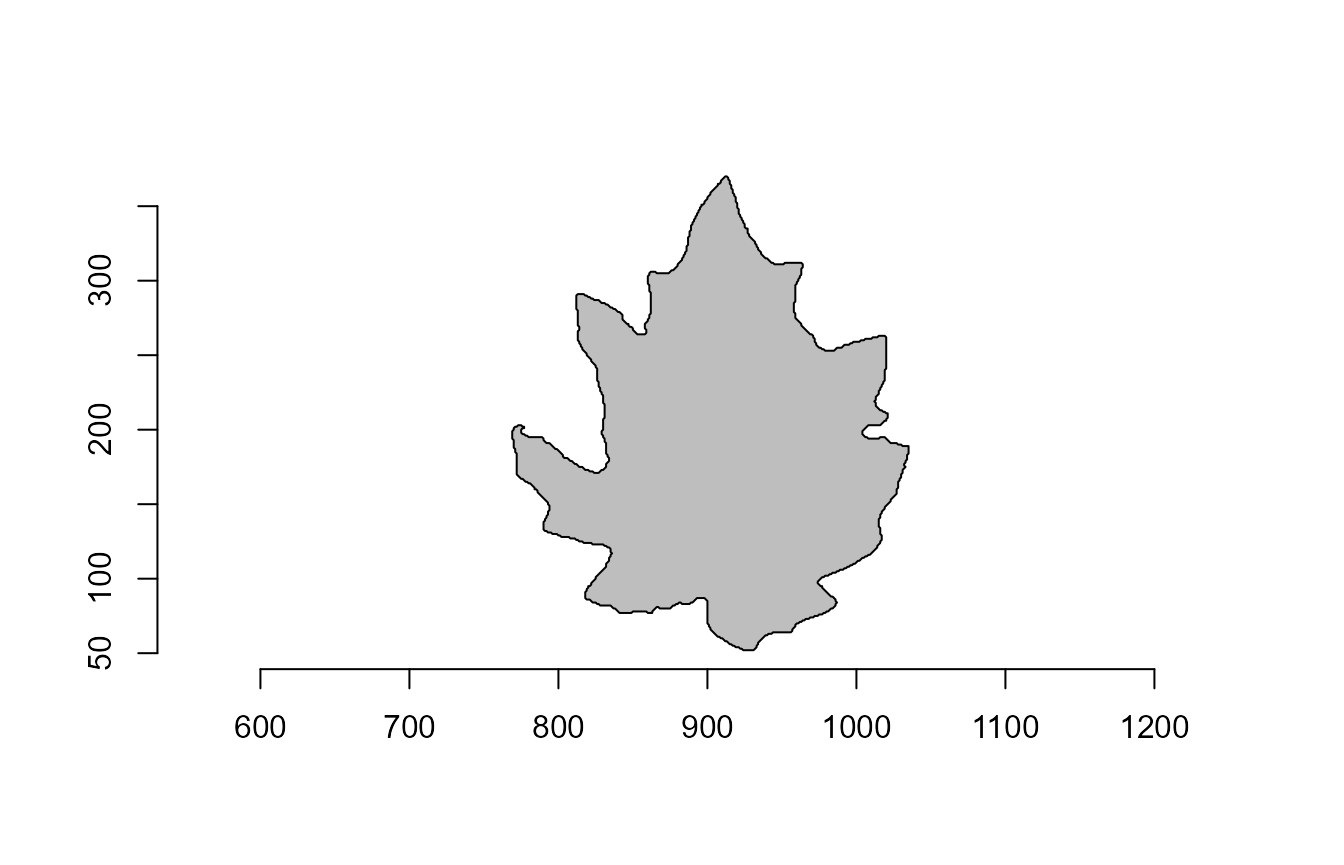### Rotate polygons

poly_rotate() can be used to rotate the polygon coordinates by a angle (0-360 degrees) in the trigonometric direction (anti-clockwise).

rot <- poly_rotate(o2, angle = 45)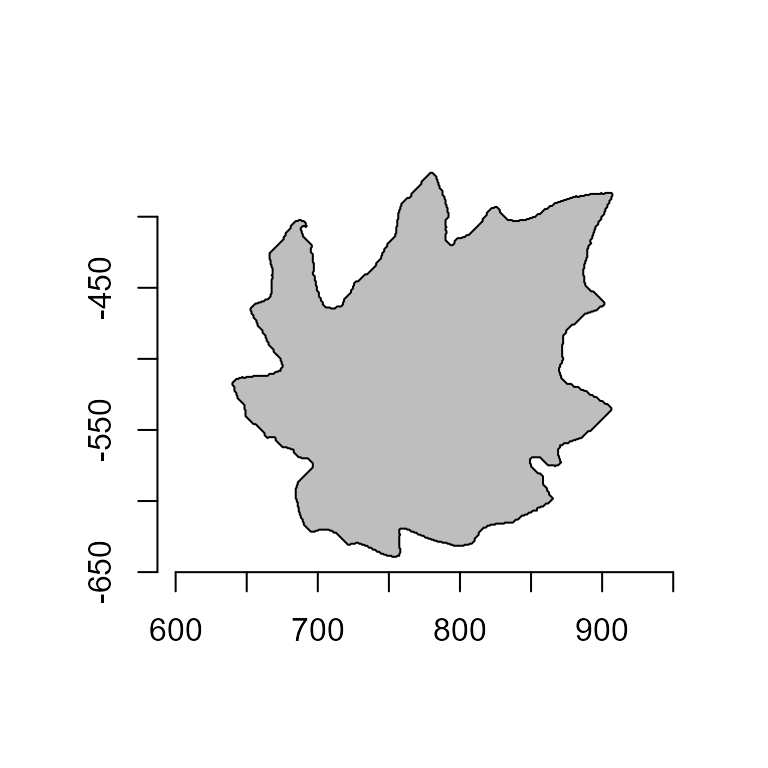### Flip polygons

poly_flip_x() and poly_flip_y() can be used to flip shapes along the x and y axis, respectively.

flip <- list(
fx = poly_flip_x(o2),
fy = poly_flip_y(o2)
)
plot_polygon(flip, merge = FALSE, aspect_ratio = 1)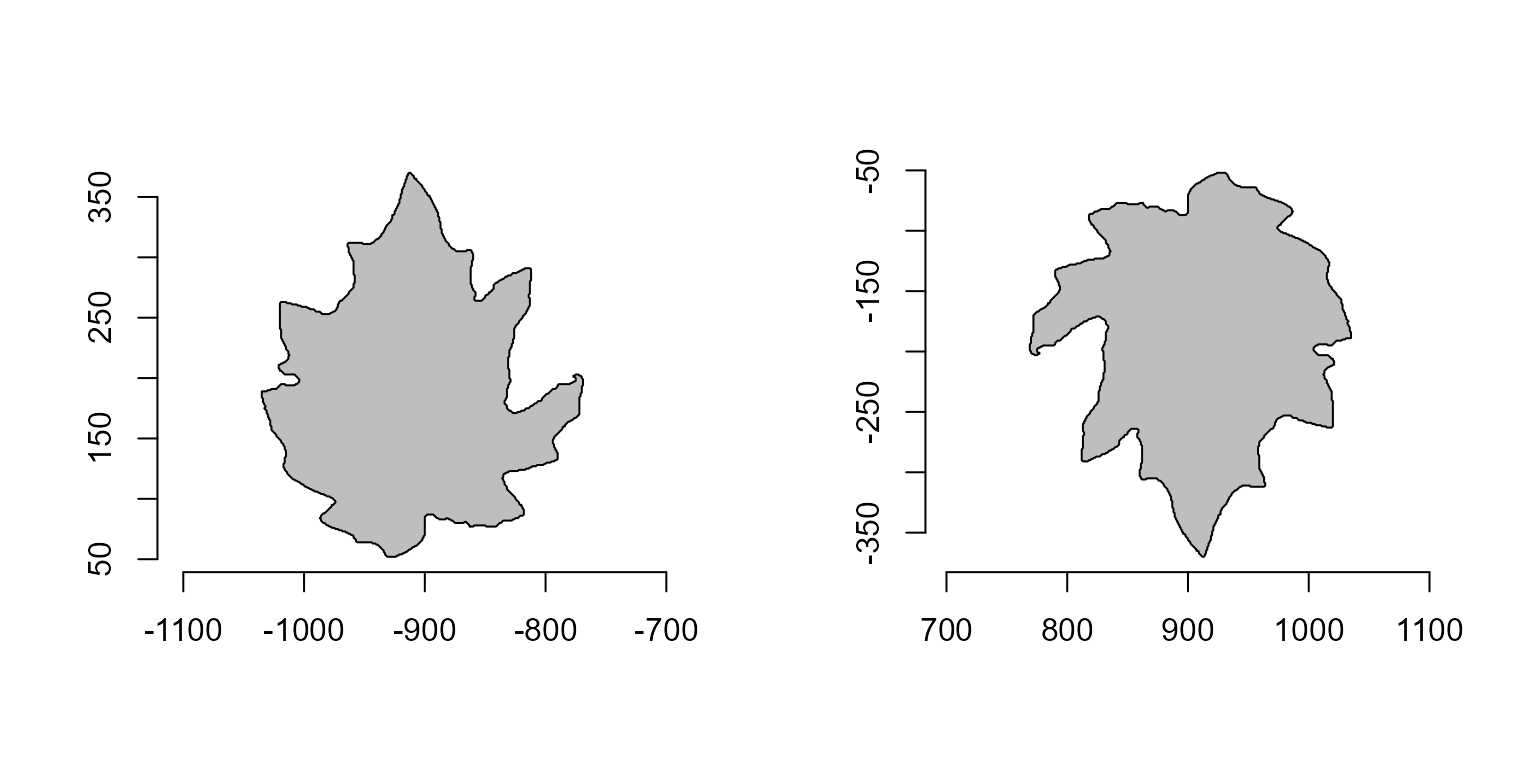### Sample points

poly_sample() samples n coordinates among existing points, and poly_sample_prop() samples a proportion of coordinates among existing.


# sample 50 coordinates
poly_sample(o2, n = 50) |> plot_polygon()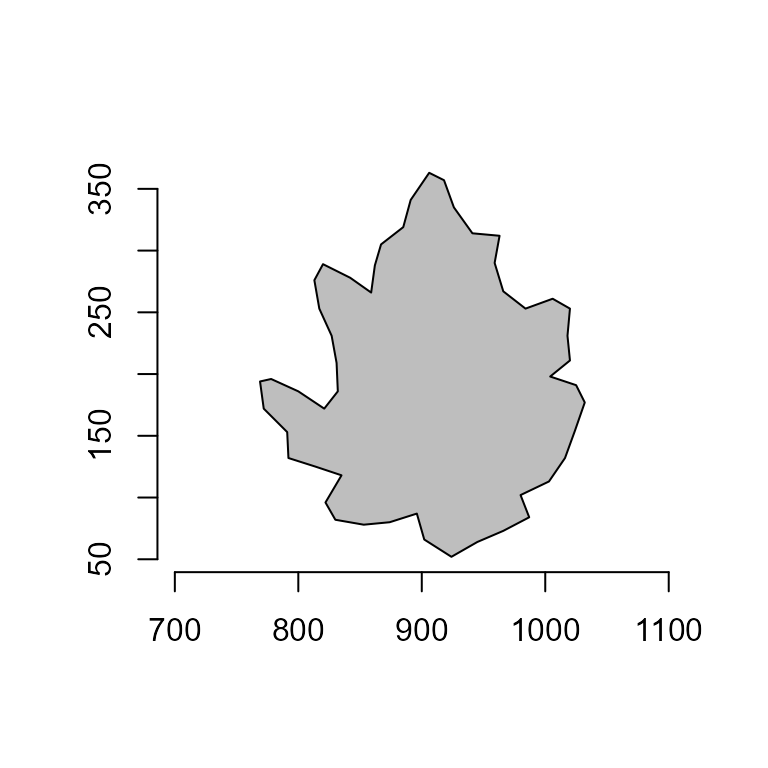# sample 10% of the coordinates
poly_sample_prop(o2, prop = 0.1) |> plot_polygon()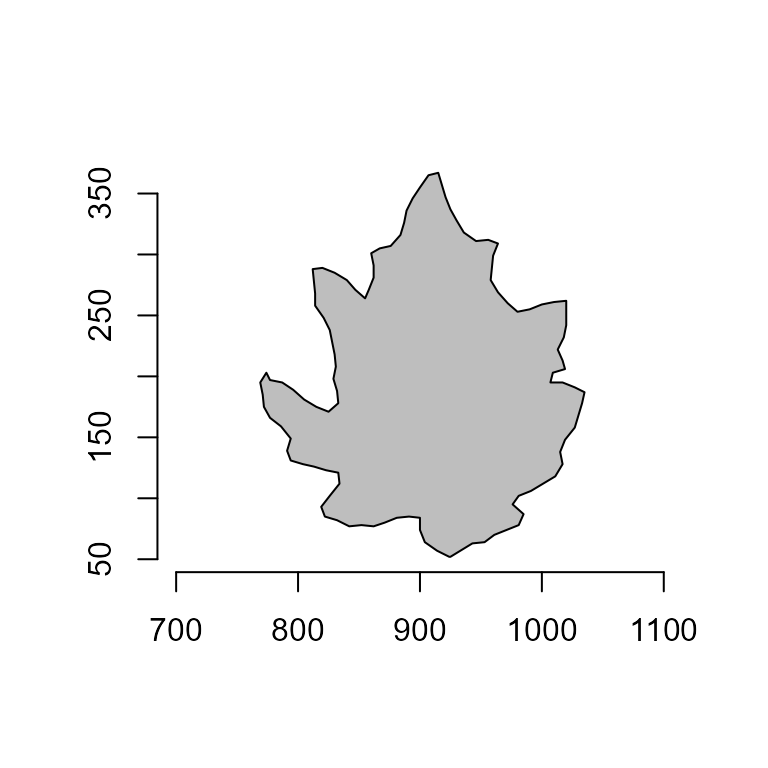### Smooth polygons

poly_smooth() smooths a polygon contour by combining sampling prop coordinate points and interpolating them using vertices vertices.

smooths <-
list(
s1 <- poly_smooth(o2, prop = 0.2, plot = FALSE),
s2 <- poly_smooth(o2, prop = 0.1, plot = FALSE),
s1 <- poly_smooth(o2, prop = 0.05, plot = FALSE)
)
plot_polygon(smooths, merge = FALSE, ncol = 3)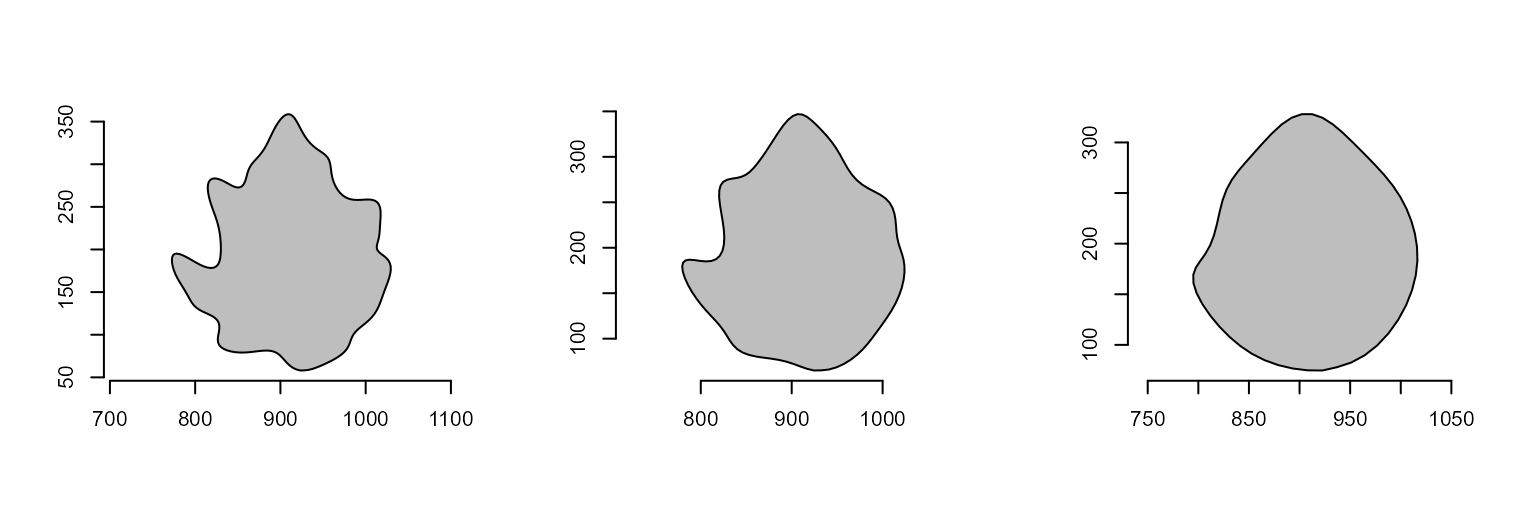### Add noise to a polygon

poly_jitter() adds a small amount of noise to a set of point coordinates. See base::jitter() for more details.

poly_jitter(o2, noise_x = 5, noise_y = 5) |> plot_polygon()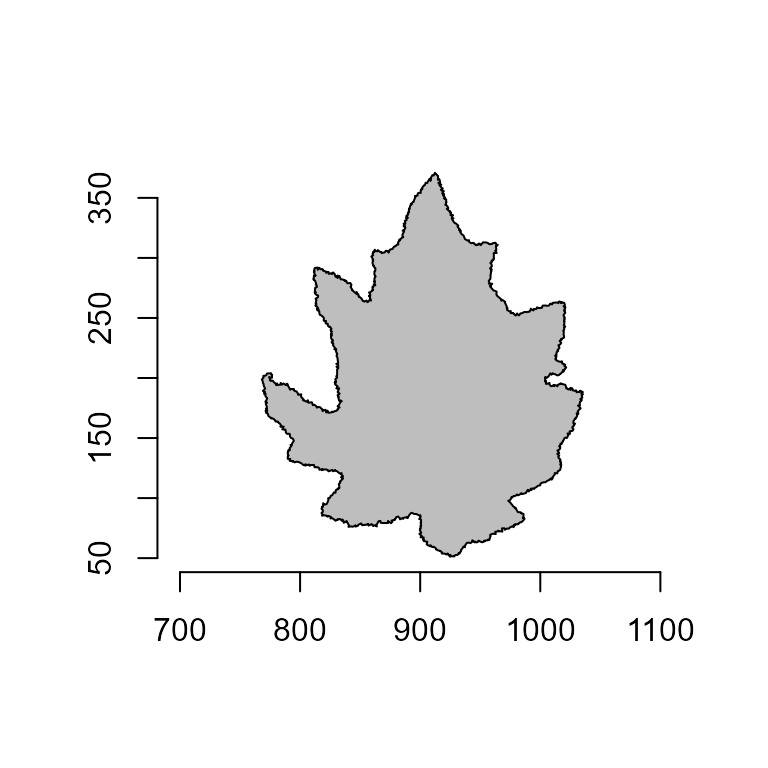## A little bit more!

At this link you will find more examples on how to use {pliman} to analyze plant images. Source code and images can be downloaded here. You can also find a talk (Portuguese language) about {pliman} here. Lights, camera, {pliman}!